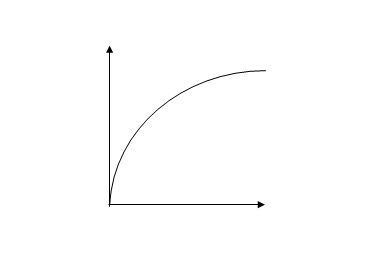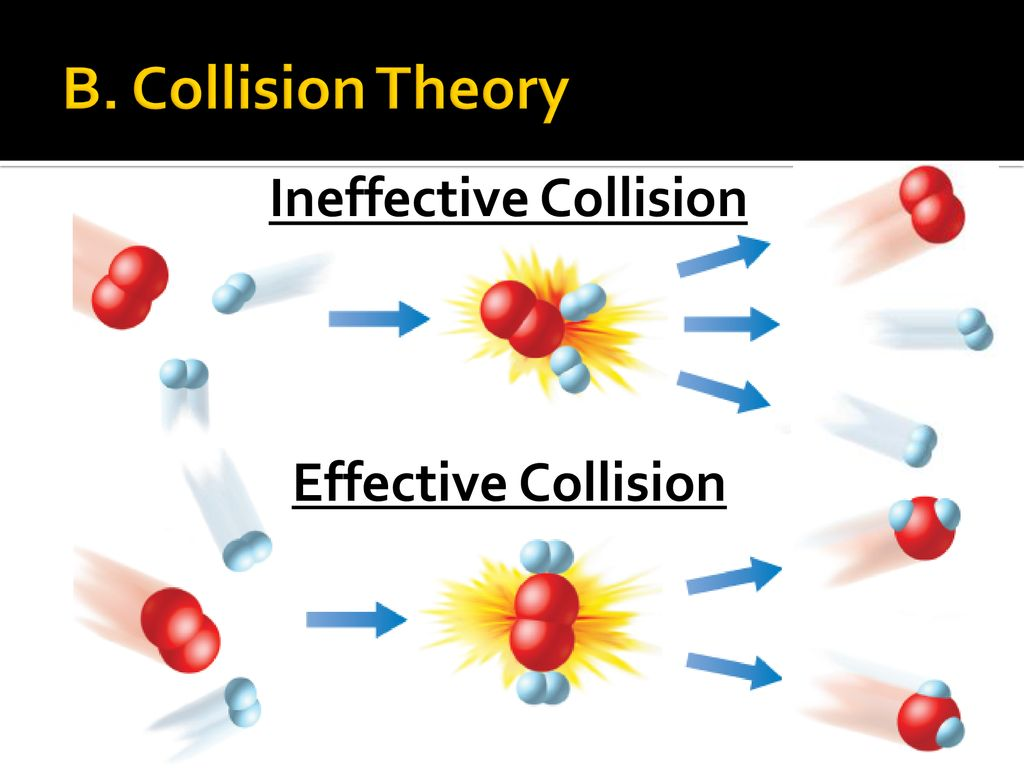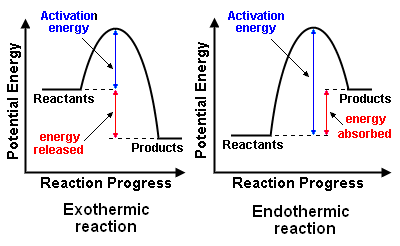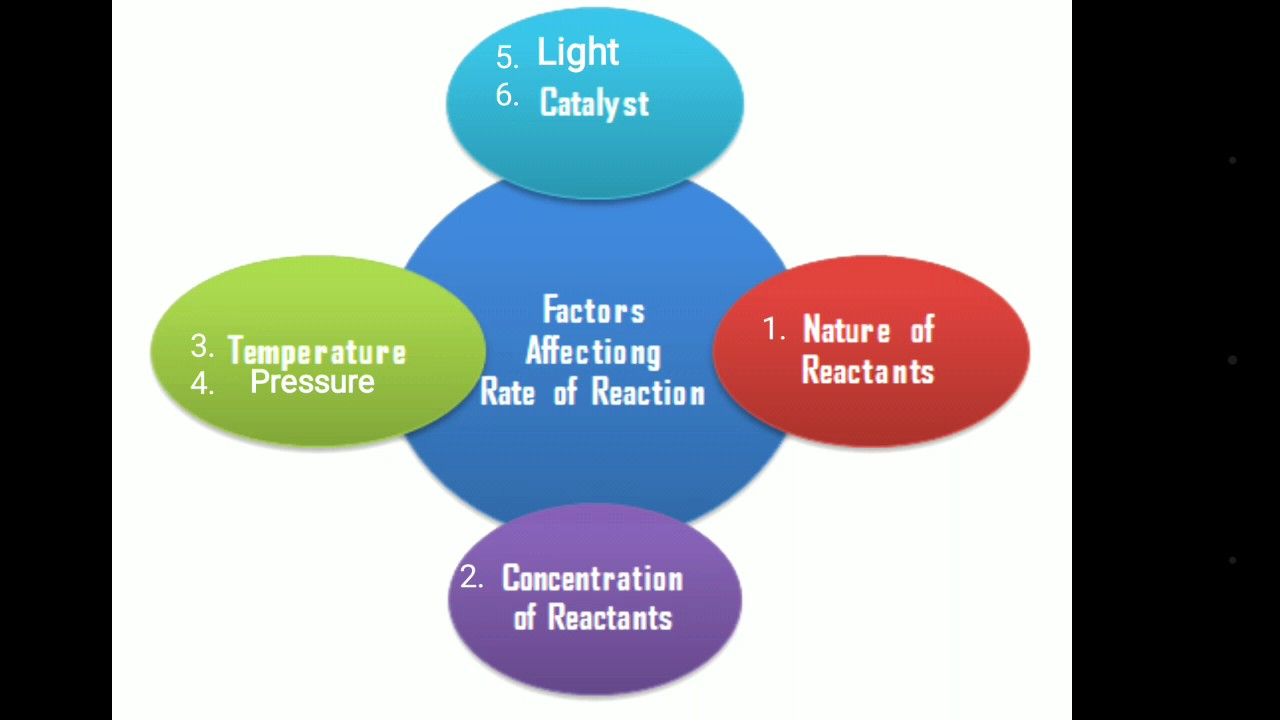# Rates of Chemical  Reaction

Welcome to SS2 Second Term!

In today’s Chemistry class, We will be discussing “Rates of Chemical Reaction”. We hope you enjoy the class!

CONTENT

• Meaning of Rate of Chemical Reaction.
• Rate Curve.
• The Collision Theory.
• Factors Affecting the Rate of Chemical Reactions#### MEANING OF RATE OF REACTION

The rate of a chemical reaction is the number of moles of reactants converted or products formed per unit time.

Usually, the rate of reaction is determined experimentally by measuring the change in concentration of one of the components in the reaction with time.

Thus,

Rate of reaction =

The unit of the rate of reaction is mol/dm-3s-1 or g dm-3s –1.

Rate of reaction can also be expressed as:

Rate of reaction =

Then the unit of rate is mols-1 or gs-1

EXAMPLE: When 0.5g of calcium trioxocarbonate (IV) was added to excess dilute hydrochloric acid, carbon (IV) oxide was evolved. The complete reaction took 5 minutes. What was the rate of reaction?

SOLUTION:

Rate of reaction =

#### WAYS OF MEASURING REACTION RATE

Concentration is one of the properties of a reaction that can change with time.

The following properties can also change with time and can thus be used to measure the rate of reaction.

1. The decrease in the mass of the reaction system
2. The volume of gaseous product
3. Amount of precipitate formed
4. Change in colour intensity
5. Change in pH
6. Change in total gas pressure

#### RATE CURVE

The rate curve is a graphical illustration of the rate of a reaction.

The following graph illustrates a rate curveFEATURES OF RATE CURVE

1. It passes through the origin. This is because there is no change in concentration or mass at the start of the reaction.
2. It steeps at first, this because the rate is fast at the beginning.
3. It becomes less steep later. This is because the rate slows down.
4. It finally becomes horizontally. This is because the reaction has reached the endpoints.

The following can be determined from the rate curve

1. The average rate of reaction

Average rate =

1. The rate at a particular instant during the reaction

The rate at instant = Gradient at a point on the curve

When the rate of reaction has a direct variation with concentration, then

Rate of reaction   α [Concentration of A]

R α [A]

R = k[A]

Where k is called the Rate constant

SELF EVALUATION

1. What is rate of reaction?
2. State two ways of measuring reaction rate

COLLISION THEORY

The collision theory states that for a chemical reaction to occur the reactant particles must collide and they must collide with a certain minimum amount of energy known as activation energy.

Reacting particles are in continuous motion, thus they possess energy and they also collide with one another. Not all collisions result in a chemical reaction. Collisions, which result in a chemical reaction, are called EFFECTIVE COLLISIONS. The minimum amount of energy required by reacting particles for chemical reaction to occur is called ACTIVATION ENERGY. Activation energy is the ENERGY BARRIER the reactants must overcome for reaction to occur. It is the minimum energy required for bond breaking for a chemical reaction to occur.A chemical reaction occurs only when the energy of the colliding reactant particles is equal to or more than the activation energy. Activation energy must be equal to energy barriers also for chemical reaction to occur.

Every reaction has its own energy of activation. Reactions with low activation energy have a high rate of reaction and occur spontaneously. Reactions with high activation energy have a low rate of reaction and are not spontaneous.

The graph below illustrates the concepts of the activation energy for endothermic and exothermic reactions.

From the graph, it can be seen that

1. Both exothermic and endothermic reactions generally require an initial input of energy to overcome the activation energy barriers.
2. Exothermic reactions once started to proceed without any further external energy supply e.g. burning of kerosene
3. Endothermic reactions need a  continuous supply of external energy to proceed e.g cooking of rice.SELF EVALUATION

1. State collision theory.
2. Graphically differentiate exothermic reaction from endothermic reaction.

###### FACTORS AFFECTING RATE OF REACTION

From the collision theory, it can be seen that rates of reaction depend on the following features.

1. The energy of the particle.
2. The frequency of collision of the reaction.
3. The activation energy of the reaction.These features of a chemical reaction are in turn affected by some factors, which can make them change and consequently affect the rate of reaction. These are factors that affect the rate of reactions. Some important ones are:

1. Nature of reactants.
2. Concentration / pressure (for gases) of reactants.
3. The surface area of reactants
4. The temperature of the reaction mixture
5. Presence of light
6. Presence of catalysts

To study the effect of any one of these factors on the rate of reaction all other factors must be kept constant.

EFFECT OF NATURE OF REACTANTS

If all other factors are kept constant, different substances will have a different rate of reaction with dilute HCl, for example. When dilute HCl reacts with zinc, iron and gold under the same condition, hydrogen gas is evolved fast with zinc, slow with iron and no gas evolved with gold.

The difference in the rate of reaction is due to the chemical nature of the elements as they naturally possess different amounts of energy content.

EFFECT OF CONCENTRATION OF REACTANTS

The frequency of collision among particles is high when the particles are crowded in a small space, i.e. high concentration. This leads to high effective collision and thus a high rate of reaction. An increase or decrease in the concentration of the reactants will result in a corresponding increase or decrease in effective collisions of the reactants and hence the reaction rate.

EFFECT OF SURFACE AREA OF REACTANTS

This is a very important factor to be considered when a solid is involved in a chemical reaction. Lumped solids offer a small surface area of contact for a reaction while powdered solids offer a large surface area for reaction. Rate of reaction is slow with lumped solid but high with powdered solids.

EFFECT OF TEMPERATURE

Increasing the temperature of a system can lead to an increase in reaction rate in two ways. When heat is raised, energy in the form of heat is supplied to the reactant particles, so that

1. The number of particles with energy equal to or greater than the activation energy increases.
2. The velocity of all the reactant particles increases due to the greater kinetic energy, leading to a higher frequency of collision.

As a result, the number of effective collisions increases and the reaction proceeds at a faster rate. Decreases in temperature lead to decrease rate of reactions.

EFFECT OF LIGHT

Some reactions are influenced by light. The rate of reaction is high when the intensity of the light is high, low when the intensity is low and does not proceed at all in the absence of light. Such reactions are known as photochemical reactions. Examples of photochemical reactions include.

1. The reaction between hydrogen and chlorine
2. Decomposition of hydrogen peroxide
3. Reactions between methane and chlorine
4. Photosynthesis in plant
5. Conversion of silver halides to grey metallic silver.

EFFECT OF CATALYST

A Catalyst is a substance, which alters the rate of a reaction, but itself does not undergo any change at the end of the reaction.

A positive catalyst increases the rate of reaction by lowering the activation energy of the reaction whereas, the one which increases the activation energy is known as a negative catalyst or an inhibitor.

The diagram below illustrates the energy profile for catalyzed and uncatalyzed exothermic and endothermic reactions

ENDOTHERMIC REACTION                                    EXOTHERMIC REACTION

GENERAL EVALUATION/REVISION

1. List and explain three factors that can affect the rate of reaction of the following reaction: CaCO3(s) + 2HCl(aq)→ CaCl2(aq) + H2O(l) + CO2(g)
2. In a chemical reaction, after 10seconds, 6moles from the initial concentration of 16moles of the reactant disappeared. Calculate the rate of the reaction.
3. State THREE characteristics of catalyst.
4. What is the volume in dm3 of 8g of oxygen gas at s.t.p?
1. Calculate the percentage of water in sodium trioxocarbonate (VI) heptahydrate

READING ASSIGNMENT: New School Chemistry for Senior Secondary School by O.Y.Ababio (6th edition) pages 250-261

WEEKEND ASSIGNMENT

1a.      Define rate of reaction

1. State the collision theory

2a.      Explain in terms of collision theory, how the rate of gaseous reaction is affected by an increase in pressure

b.      Give the reason why red-hot iron wool reacts more readily with oxygen than red-hot iron nail

We have come to the end of this class. We do hope you enjoyed the class?

Should you have any further question, feel free to ask in the comment section below and trust us to respond as soon as possible.

In our next class, we will be talking about Exothermic and Endothermic Reactions. We are very much eager to meet you there.

Are you a Parent? Share your quick opinion and win free 2-month Premium Subscription

### 1 thought on “Rates of Chemical  Reaction”

1.NICE NOTES

Don`t copy text!